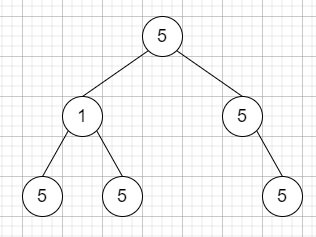# Count Univalue Subtrees in C++

C++Server Side ProgrammingProgramming

Suppose we have a binary tree; we have to count the number of uni-value subtrees. Here the Uni-value subtree indicates all nodes of the subtree have the same value.

So, if the input is like root = [5,1,5,5,5,null,5],then the output will be 4

To solve this, we will follow these steps −

• Define a function solve(), this will take node,

• if node is empty, then −

• return true

• left := solve(left of node)

• right := solve(right of node)

• if left is false or right is false, then −

• return false

• if left of node is present and val of node is not equal to value of left of node, then −

• return false

• if right of node is present and val of node is not equal to value of right of node, then −

• return false

• (increase ret by 1)

• return true

• From the main method do the following −

• ret := 0

• solve(root)

• return ret

## Example

Let us see the following implementation to get a better understanding −

Live Demo

#include <bits/stdc++.h>
using namespace std;
class TreeNode{
public:
int val;
TreeNode *left, *right;
TreeNode(int data){
val = data;
left = NULL;
right = NULL;
}
};
void insert(TreeNode **root, int val){
queue<TreeNode*> q;
q.push(*root);
while(q.size()){
TreeNode *temp = q.front();
q.pop();
if(!temp->left){
if(val != NULL)
temp->left = new TreeNode(val);
else
temp->left = new TreeNode(0);
return;
}
else{
q.push(temp->left);
}
if(!temp->right){
if(val != NULL)
temp->right = new TreeNode(val);
else
temp->right = new TreeNode(0);
return;
}
else{
q.push(temp->right);
}
}
}
TreeNode *make_tree(vector<int< v){
TreeNode *root = new TreeNode(v);
for(int i = 1; i<v.size(); i++){
insert(&root, v[i]);
}
return root;
}
class Solution {
public:
int ret;
bool solve(TreeNode* node){
if (!node || node->val == 0)
return true;
bool left = solve(node->left);
bool right = solve(node->right);
if (!left || !right)
return false;
if (node->left && node->left->val != 0 && node->val != node->left->val)
return false;
if (node->right && node->right->val != 0 && node->val != node->right->val)
return false;
ret++;
return true;
}
int countUnivalSubtrees(TreeNode* root){
ret = 0;
solve(root);
return ret;
}
};
main(){
Solution ob;
vector<int< v = {5,1,5,5,5,NULL,5};
TreeNode *root = make_tree(v);
cout << (ob.countUnivalSubtrees(root));
}

## Input

{5,1,5,5,5,NULL,5}

## Output

4